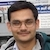# NAEST 2019 Screening Test: Opening a Bottle CapBy

A bottle's cap is closed by rotating it over the bottle's orifice (mouth). The rotation engages threads between the cap and the bottle. The frictional force/torque between the cap and the bottle holds the two together. To open the cap, torque should be applied in a direction that disengages the threads. The magnitude of the applied torque is greater than the frictional torque. The video shows a person trying to open the cap. In case 1, he failed to open the cap. In case 2, he succeeds with the help of a cloth.

Question 1: CASE 1 : Mark the correct statement(s)

1. There is a frictional force between the fingers and the cap but there is no frictional force between the bottle and the cap.
2. Torque of the friction by the fingers on the cap is clockwise (as seen from the top).
3. The torque of the friction by the bottle on the cap is clockwise (as seen from the top).
4. The torque by the fingers on the cap is anticlockwise (as seen from the top).

Question 2: CASE 2: Let the magnitude of the frictional force applied by the fingers in CASE-1 on the cap at each contact point be $F_1$. Let the magnitude of the frictional force applied by the cloth in CASE-2 on the cap at each contact point be $F_2$. Mark the correct statement(s).

1. $F_1=F_2$
2. $F_1< F_2$
3. $F_1 > F_2$
4. The resultant force on the cap during the period it is opening is zero.

Solution: In this discussion, clockwise and anticlockwise directions are defined when we look at the bottle from outside (from the top).

The action of cap's opening produces two opposing torques on the cap. Let us focus on two specific points P and Q (see figure). The point P lies at the interface of fingers and the cap and the point Q lies at the interface of the cap and the bottle.At point P, the fingers tends to slide over the cap in the anticlockwise direction. The frictional force by the cap on the fingers opposes this tendency. By Newton's third law, the frictional force by the fingers on the cap ($F_1$) is as shown in the figure. The torque $\tau_1$ due to $F_1$ about the centre of the cap is anticlockwise. Thus, option (D) of question 1 is correct.

At point Q, the cap tends to slide over the bottle in the anticlockwise direction. To oppose this tendency, the frictional force $F_2$ on the cap by the bottle is as shown in the figure. The torque $\tau_2$ due to $F_2$ about the centre of the cap is clockwise.

The cap gets open if $\tau_1$ is greater than $\tau_2$. This requires $F_1$ to be greater than $F_2$ (neglecting thickness of the cap). Thus, option (C) of question 2 is correct. Note that the net horizontal force on the cap is zero because it is not accelerating in the horizontal direction.

After the initial struggle, the cap opens up smoothly. The centre of mass of the cap moves upwards. Thus, there is a net force on the cap in the upward direction.

I encourage you to analyse the limiting value of the frictional force. Generally, you press the cap with the fingers to increase normal reaction. This increases the frictional force $F_1$ and the torque $\tau_1$.

In case 2, the cloth helps in opening the cap. The cloth has more coefficient of friction as compared to fingers. This increases the frictional force by the fingers on the cap.